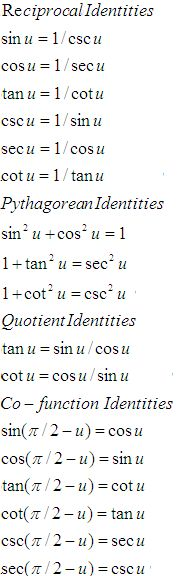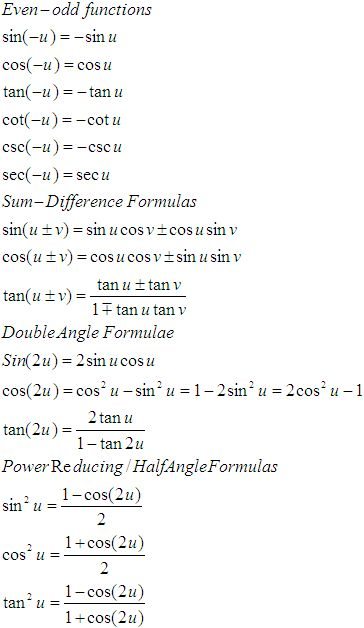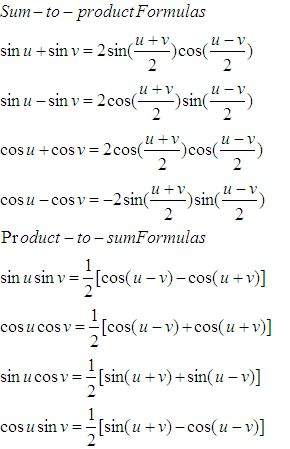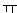Name: ___________________Date:___________________

 Email us to get an instant 20% discount on highly effective K-12 Math & English kwizNET Programs!

### High School Mathematics - 210.18 Trignometry Review

 Function 0o 30° 45° 60° 90° sin Ö0/2 Ö1/2 Ö2/2 Ö3/2 Ö4/2 cos Ö4/2 Ö3/2 Ö2/2 Ö1/2 0 tan 0 Ö3/3 1 Ö3 undefined sec 1 2Ö3/3 Ö2 2 undefined csc undefined 2 Ö2 2Ö3/3 1 cot undefined Ö3 1 Ö3/3 0

 sin cos tan 0 0 1 0 90 1 0 infinity 180 0 -1 0 270 -1 0 infinityFunctions of angles in all quadrants in terms of those in quadrant 1
 -A 900 ± A P/2 ± A 1800 ± A P ± A 2700 ± A 3P/2 ± A 3600 ± A 2P ± A sin -sinA cos A ±sin A -cosA ±sin A cos cos A ±sin A -cos A ±sin A cos A tan -tan A ±cot A ± tan A ±cot A ±tan A csc -csc A sec A ± csc A -sec A ±csc A sec sec A ± csc A -sec A ±csc A sec A cot -cot A ± tan A ± cot A ±tan A ± cot AName: ___________________Date:___________________

### High School Mathematics - 210.18 Trignometry Review

 Q 1: The angle of elevation of the top of a tower which is yet incomplete at a point 120 metres from its base is 45o. How much higher should it be raised so that the elevation at the same point may become 60o?100 metres36.85 metres87.84 metres Q 2: In triangle ABC, a = 8.4 cm, b = 7.6 cm and c = 9.2 cm. Calculate the area of triangle ABC.50 sq. cm30 sq cm45 sq cm Q 3: The angle of elevation of the top of a tower from a point 60m from its foot is 30o. Find the height of the tower.100 m20 m2031/2 m Q 4: Find the radian measure of 15o./4/6/12 Q 5: The sines of the angles of a triangle are in the ratio of 4:5:6, find the ratio of the cosines of the angles.2:3:44:5:212:9:2 Q 6: Find the degree measure of 3/4.42057l18ll45o35o Q 7: If the shadow of a tower is 30 metres, when the sun's altitude is 30o, what is the length of the shadow when the sun's altitude is 60o?200 metres100 metres240 metres Q 8: The angles of a triangle ABC are in AP and b:c = 31/2:21/2. Find angle A.60 degrees45 degrees75 degrees Question 9: This question is available to subscribers only! Question 10: This question is available to subscribers only!